Instantly share code, notes, and snippets.gizmaa/Plot_Examples.md Last active Jun 2, 2019

Various Julia plotting examples using PyPlot

Plotting

Last Update: May 13, 2019
Offline Version

PyPlot

Translating

Translating PyPlot code from Python to Julia can be difficult so here are a few examples comparing Python code with its Julia equivalent.

# Python
ax.set_ylim([-30, 10])
ax.spines['right'].set_color('none')
ax.spines['top'].set_color('none')

Source: Axis Boundary Color

# Julia
ax.set_ylim([-30,10])
ax.spines["top"].set_color("none") # Remove the top axis boundary
ax.spines["right"].set_color("none") # Remove the right axis boundary

The above example looked at settings of plot components. The next example will call matplotlib itself.

# Python
from matplotlib.dates import MonthLocator, WeekdayLocator, DateFormatter
majorformatter = DateFormatter("%d.%m.%Y")
minorformatter = DateFormatter("%H:%M")
majorlocator = DayLocator(interval=1)
minorlocator = HourLocator(byhour=(8,16)) # Not sure about this one

Source: Modified from this forum post by Nat Wilson and this matplotlib example.

# Julia
majorformatter = matplotlib.dates.DateFormatter("%d.%m.%Y")
minorformatter = matplotlib.dates.DateFormatter("%H:%M")
majorlocator = matplotlib.dates.DayLocator(interval=1)
minorlocator = matplotlib.dates.HourLocator(byhour=(8, 16))

# After an axis exists
ax1.xaxis.set_major_formatter(majorformatter)
ax1.xaxis.set_minor_formatter(minorformatter)
ax1.xaxis.set_major_locator(majorlocator)
ax1.xaxis.set_minor_locator(minorlocator)

Important Note

The easiest way of doing a quick plot is to simply type it into the REPL (command line) but by default interactive mode might be "off". This means that when you create a figure, figure(), nothing will appear except for the object type in the REPL, PyPlot.Figure(PyObject .... The command plt[:show]() will make the figure visible but also make the REPL temporarily unusable until all figures are closed.

Changing interactive mode to "on" is as simple as running ion(). Plots will be visible and the REPL will still be usable. It will only last for the current session though. Add it to the .juliarc.jl file to make it "on" by default. It can also be turned "off" by running ioff(). If IJulia fails to plot inline try adding gcf() after the plot.

Depending on the editor you are using this may be undesirable. In one mode IJulia may plot inline whereas the other may plot to a window.

Basic Plot

Most of the basic commands in PyPlot are very similar to Matlab.

p = plot(x,y)
xlabel("X")
ylabel("Y")
grid("on")

The first noticable change is in the plotting command when non-default values are used.

p = plot_date(x,y,linestyle="-",marker="None",label="Base Plot") # Basic line plot

Instead of "linestyle","-" it uses linestyle="-" for parameters.

Plot Annotation

(IJulia, Code)The following command will point an arrow at a point and label the arrow.

annotate("Look, data!",
xy=[x;y,# Arrow tip
xytext=[x+dx;y+dy], # Text offset from tip
xycoords="data", # Coordinates in in "data" units
arrowprops=["facecolor"=>"black"]) # Julia dictionary objects are automatically converted to Python object when they pass into a PyPlot function

It's important to note that in Python the arrowprops would look like this: arrowprops=dict(arrowstyle="->"). Dictionary definitions look like arrowprops=["facecolor"=>"black"] in Julia.

LaTeX can be used by putting an L in front of LaTeX code, L"$\int x = \frac{x^2}{2} + C$".

annotate(L"$\int x = \frac{x^2}{2} + C$",
xy=[1;0],
xycoords="axes fraction",
xytext=[-10,10],
textcoords="offset points",
fontsize=30.0,
ha="right",
va="bottom")

Time Customization

(IJulia,Code)

The formatting preparation is accomplished by calling the formatters within Matplotlib.

majorformatter = matplotlib.dates.DateFormatter("%d.%m.%Y")
minorformatter = matplotlib.dates.DateFormatter("%H:%M")
majorlocator = matplotlib.dates.DayLocator(interval=1)
minorlocator = matplotlib.dates.HourLocator(byhour=(8, 16))

They are then applied to the specific axis, the handle of which is called ax1 in this case.

ax1.xaxis.set_major_formatter(majorformatter)
ax1.xaxis.set_minor_formatter(minorformatter)
ax1.xaxis.set_major_locator(majorlocator)
ax1.xaxis.set_minor_locator(minorlocator)Subplots

(IJulia, Code)

subplot(YXN), Y = number of columns, X = number of rows, N = number of axis being created

The number, N, of a grid of axes starts in the upper left (1), and goes right then down. The second axis of a 2x2 grid is the upper right axis.

subplot(313) # Create the third plot of a 3x1 group of subplots

suptitle("3x1 Subplot") # Supe title, title for all subplots combinedPolar and Windrose Plot

(IJulia, Code)

ax = axes(polar="true") # Create a polar axis

# Optional changes
ax.set_thetagrids(collect(0:dtheta:360-dtheta)) # Show grid lines from 0 to 360 in increments of dtheta
ax.set_theta_zero_location("N") # Set 0 degrees to the top of the plot
ax.set_theta_direction(-1) # Switch to clockwise
fig.canvas.draw() # Update the figureHistogram

(IJulia, Code)

h = plt.hist(x,nbins) # Histogram, PyPlot.plt required to differentiate with conflicting hist commandBar Plot

(IJulia, Code)

b = bar(x,y,color="#0f87bf",align="center",alpha=0.4)
b = barh(x,y,color="#0f87bf",align="center",alpha=0.4)Errorbar Plot

(IJulia, Code)

errorbar(x, # Original x data points, N values
y, # Original y data points, N values
yerr=errs, # Plus/minus error ranges, Nx2 values
fmt="o") # FormatInexact Plot

(IJulia, Code)

The IJulia example does not properly apply all the formatting as the terminal version does.

xkcd() # Set to XKCD mode, based on the comic (hand drawn)
# Plot everythingPie Chart

(IJulia, Code)

p = pie(sizes,labels=labels,shadow=true,startangle=90,explode=explode,colors=colors,autopct="%1.1f%%",textprops=font)Scatter Plot

(IJulia, Code)

scatter(x,y,s=areas,alpha=0.5)Box Plot

(IJulia, Code)

boxplot(data, # Each column/cell is one box
notch=true, # Notched center
whis=0.75, # Whisker length as a percent of inner quartile range
widths=0.25, # Width of boxes
vert=false, # Horizontal boxes
sym="rs") # Symbol color and shape (rs = red square)Major and Minor Ticks

(IJulia, Code)

###########################
#  Set the tick interval  #
###########################
Mx = matplotlib.ticker.MultipleLocator(20) # Define interval of major ticks
f = matplotlib.ticker.FormatStrFormatter("%1.2f") # Define format of tick labels
ax.xaxis.set_major_locator(Mx) # Set interval of major ticks
ax.xaxis.set_major_formatter(f) # Set format of tick labels

mx = matplotlib.ticker.MultipleLocator(5) # Define interval of minor ticks
ax.xaxis.set_minor_locator(mx) # Set interval of minor ticks

My = matplotlib.ticker.MultipleLocator(0.5) # Define interval of major ticks
ax.yaxis.set_major_locator(My) # Set interval of major ticks

my = matplotlib.ticker.MultipleLocator(0.1) # Define interval of minor ticks
ax.yaxis.set_minor_locator(my) # Set interval of minor ticks

#########################
#  Set tick dimensions  #
#########################
ax.xaxis.set_tick_params(which="major",length=10,width=2,labelsize=16)
ax.xaxis.set_tick_params(which="minor",length=5,width=2)

fig.canvas.draw() # Update the figureMulti-axis Plot

(IJulia, Code)

################
#  Other Axes  #
################
new_position = [0.06;0.06;0.77;0.91] # Position Method 2
ax.set_position(new_position) # Position Method 2: Change the size and position of the axis

ax2 = ax.twinx() # Create another axis on top of the current axis
font2 = Dict("color"=>"purple")
ylabel("Right Axis",fontdict=font2)
p = plot(x,y2,color="purple",linestyle="-",marker="o",label="Second") # Plot a basic line
ax2.set_position(new_position) # Position Method 2
setp(ax2.get_yticklabels(),color="purple") # Y Axis font formatting

ax3 = ax.twinx() # Create another axis on top of the current axis
ax3.spines["right"].set_position(("axes",1.12)) # Offset the y-axis label from the axis itself so it doesn't overlap the second axis
font3 = Dict("color"=>"green")
ylabel("Far Right Axis",fontdict=font3)
p = plot(x,y3,color="green",linestyle="-",marker="o",label="Third") # Plot a basic line
ax3.set_position(new_position) # Position Method 2
setp(ax.get_yticklabels(),color="green") # Y Axis font formatting

axis("tight")

# Enable just the right part of the frame
ax3.set_frame_on(true) # Make the entire frame visible
ax3.patch.set_visible(false) # Make the patch (background) invisible so it doesn't cover up the other axes' plots
ax3.spines["top"].set_visible(false) # Hide the top edge of the axis
ax3.spines["bottom"].set_visible(false) # Hide the bottom edge of the axis

fig.canvas.draw() # Update the figureAxis Placement

(IJulia, Code)

ax.spines["top"].set_visible(false) # Hide the top edge of the axis
ax.spines["right"].set_visible(false) # Hide the right edge of the axis
ax.spines["left"].set_position("center") # Move the right axis to the center
ax.spines["bottom"].set_position("center") # Most the bottom axis to the center
ax.xaxis.set_ticks_position("bottom") # Set the x-ticks to only the bottom
ax.yaxis.set_ticks_position("left") # Set the y-ticks to only the left
ax2.spines["top"].set_visible(false) # Hide the top edge of the axis
ax2.spines["right"].set_visible(false) # Hide the right edge of the axis
ax2.xaxis.set_ticks_position("bottom")
ax2.yaxis.set_ticks_position("left")
ax2.spines["left"].set_position(("axes",-0.03)) # Offset the left scale from the axis
ax2.spines["bottom"].set_position(("axes",-0.05)) # Offset the bottom scale from the axisSurface and Contour Plots

(IJulia, Code)

Thanks to Daniel Høegh for providing this example.

plot_surface(xgrid, ygrid, z, rstride=2,edgecolors="k", cstride=2,
cmap=ColorMap("gray"), alpha=0.8, linewidth=0.25)
contour(xgrid, ygrid, z, colors="black", linewidth=2.0)
ax.label(cp, inline=1, fontsize=10)Line Collection

(IJulia, Code)

Thanks to David P. Sanders for this example.

# First Segment Group
xs = [1.,3.,5.,0.]
ys = [2.,4.,.06,0.]
lines = Any[collect(zip(xs,ys))]
# lines = [(1.0,2.0),(3.0,4.0),(5.0,0.06),(0.0,0.0)]

# Second Segment Group
xs = [3.,4]
ys = [5.,6]
push!(lines,collect(zip(xs,ys)))

# Third Segment Group
xs = [8.,2]
ys = [2.,4]
push!(lines,collect(zip(xs,ys)))

##############
##  Colors  ##
##############
# Line segments will be plotted with the following order of colors and will
# cycle back to the beginning of the array when it has gone through all of them
c = Vector{Int}[[1,0,0],[0,1,0],[0,0,1]]

# Assemble everything into a LineCollection
line_segments = matplotlib.collections.LineCollection(lines,colors=c)

############
##  Plot  ##
############
fig = figure("Line Collection Example",figsize=(10,10))
ax = PyPlot.axes()
axis("image")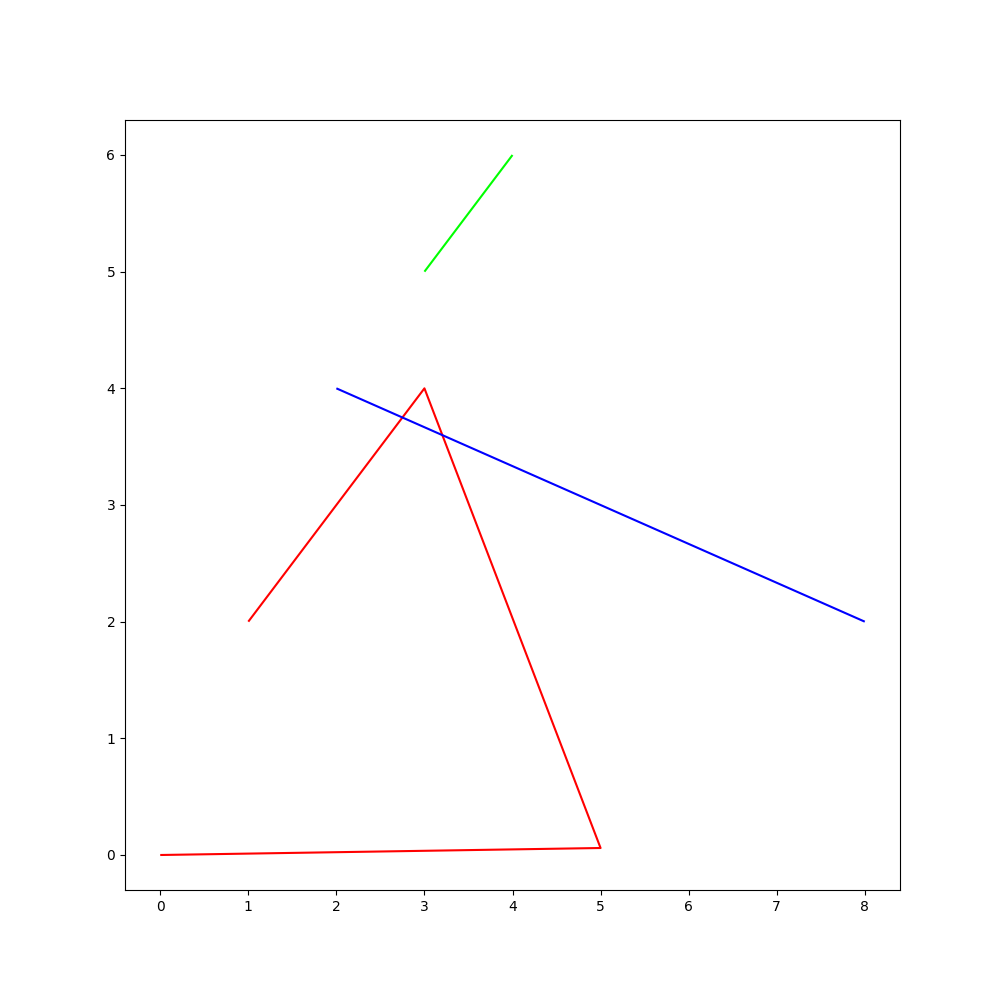3D Surf Plot

(IJulia, Code)

Thanks to feima0011 for this example.

surf(x,y,z,facecolors=colors)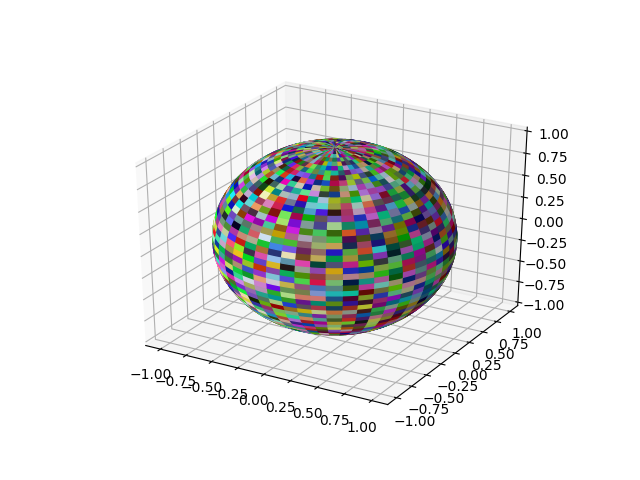Quiver Plot

(IJulia, Code)

q = quiver(X,Y,U,V)
ax = gca()
ax.quiverkey(q,X=0.07,Y = 0.05, U = 10,coordinates="figure", label="Quiver key, length = 10",labelpos = "E")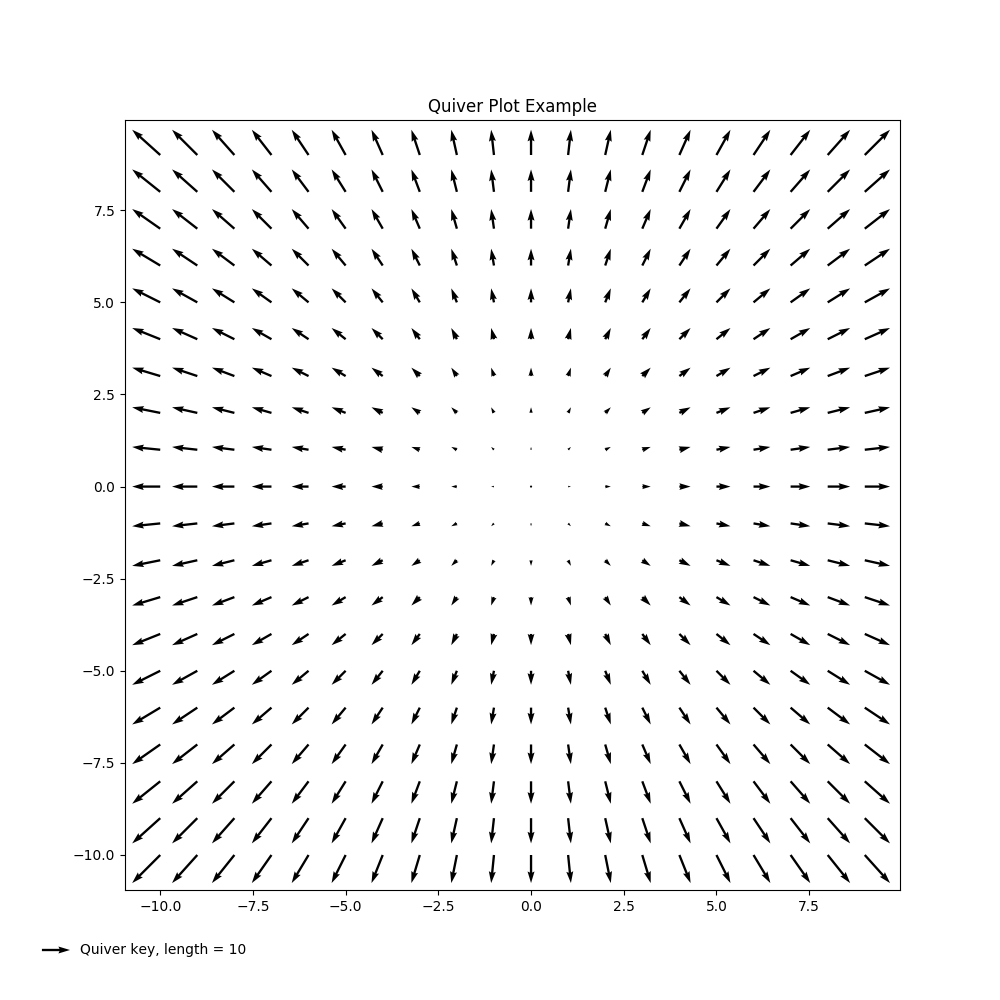Stream Plot

(IJulia, Code)

streamplot(X,Y,U,V)
streamplot(X,Y,U,V,color=U,linewidth=2,cmap=PyPlot.cm[:autumn])
lw = 5 .* speed ./ maximum(speed) # Line Widths
streamplot(X,Y,U,V,density=0.6,color="k",linewidth=lw)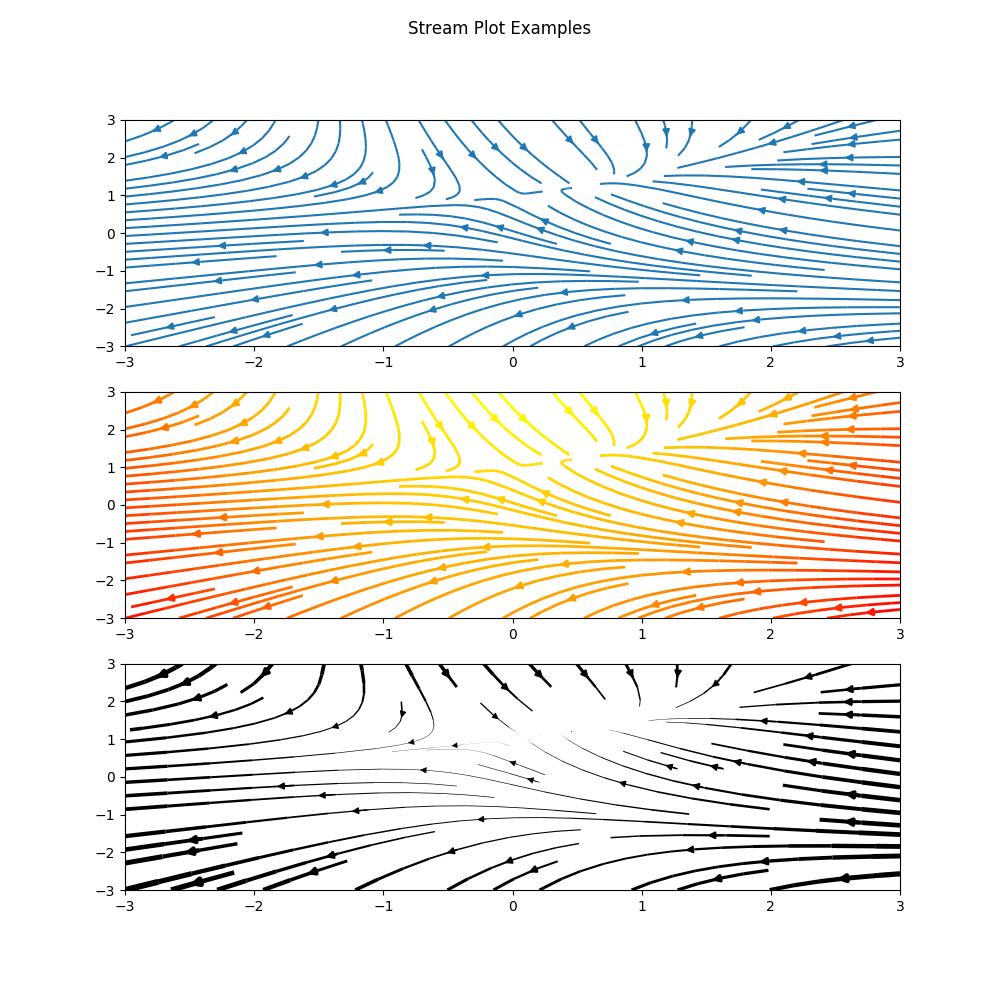Broken Axis Subplot

(IJulia, Code)

Thanks to Ian Butterworth for providing this example.

axes_grid1 = pyimport("mpl_toolkits.axes_grid1")
divider = axes_grid1.make_axes_locatable(ax)
fig.add_axes(ax2)
ax.spines["top"].set_visible(false)
ax2.spines["bottom"].set_visible(false)
# Upper Line Break Markings
d = 0.015  # how big to make the diagonal lines in axes coordinates
ax2.plot((-d, +d), (-d, +d), transform=ax2.transAxes, color="k", clip_on=false,linewidth=0.8)        # Left diagonal
ax2.plot((1 - d, 1 + d), (-d, +d), transform=ax2.transAxes, color="k", clip_on=false,linewidth=0.8)  # Right diagonal

# Lower Line Break Markings
ax.plot((-d, +d), (1 - d, 1 + d), transform=ax.transAxes, color="k", clip_on=false,linewidth=0.8)  # Left diagonal
ax.plot((1 - d, 1 + d), (1 - d, 1 + d), transform=ax.transAxes, color="k", clip_on=false,linewidth=0.8)  # Right diagonal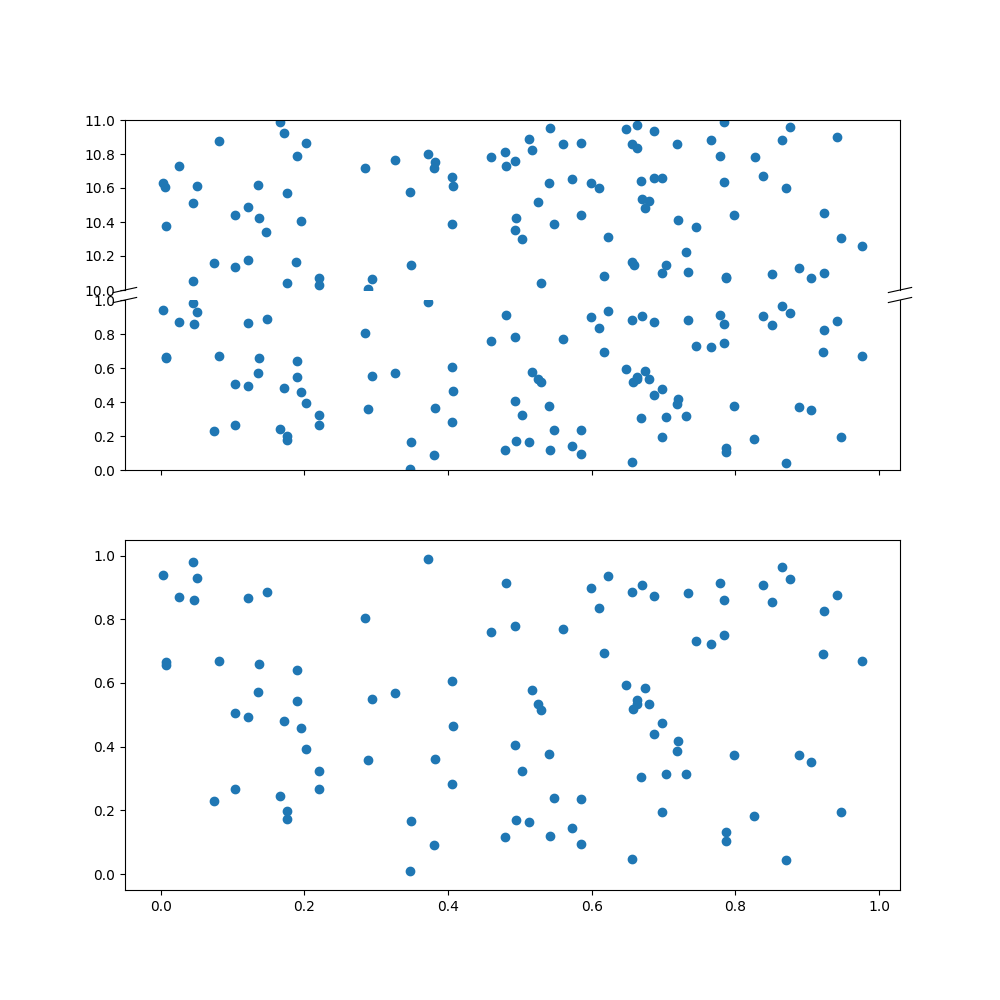floswald commented Jul 15, 2014

 this is great! thanks so much!

jstac commented Sep 10, 2014

 This has been extremely helpful. Thank you!
Owner Author

gizmaa commented Nov 28, 2014

 Everything has been updated and tested for Julia 0.3.2. I also added a new section on plotting against a custom time format.

GilMoretto commented Dec 3, 2014

 Wonderful! Thanks a lot. Do you know where to find examples translated to Julia for plot3D and surface3D? Merci bien, Gil

dhoegh commented Dec 3, 2014

 I have a surface_plot i helped a guy with in https://groups.google.com/forum/m/?fromgroups#!topic/julia-users/eVtZdp3htTM Edit: The code is now included in the gist.
Owner Author

gizmaa commented Dec 12, 2014

 An IJulia version is now available in the repository. It can be viewed online through the NBViewer or downloaded for offline use. All of the files have been renamed for consistency.

michaellindon commented Sep 19, 2015

 I think x and y have been confused in the surface and contour example. Plotting a bivariate normal with identity covariance matrix masks this.

benavoli commented Dec 1, 2015

 Can you add an example about how to change fontsize in pie chart?

uozden commented Dec 16, 2015

 Thank you very much. Very helpful indeed.

Kelvyn88 commented Jan 28, 2016

 This is the best!!!! Thank you a lot!

crunch3 commented Feb 1, 2016

 Is it still possible to get several curves plotted on the same canvas? If so, can you post some example code?
Owner Author

gizmaa commented Mar 7, 2016

 Image links updated so that they work. @benavoli, off hand I don't know how to change the pie chart font sizes but I'll take a look at it. @crunch3, what do you mean? Multiple plots on the same axis is easy. Simply plot another curve and it should be added to the last created axis. It's not like Matlab in this case in that hold("on") is not necessary. Thomas Breloff (@tbreloff)has a great Read The Docs page on Julia plotting as well for people looking for additional references. He has examples that I don't have and I have ones that he doesn't have (at least as of this writing). He also has sections on other plotting packages such as Gadfly and Winston among others.

floswald commented Mar 8, 2016

 @gizmaa, i found knowing some basic window manipulation very useful. things like PyPlot.close() # close all plot windows fig,axes = subplots(1,2) # create figure and axes ax = axes # operate on subplot 1 ax[:plot](1:10,rand(10),color="red") ax[:plot](1:10,rand(10),color="green") ax = axes # operate on subplot 2 ax[:plot](1:10,ones(10),color="yellow") ax[:plot](1:10,1:10,color="black") fig[:canvas][:set_window_title]("title of window") fig[:canvas][:draw]() # update the figure to draw if you're doing this in the REPL
Owner Author

gizmaa commented Mar 8, 2016

 @benavoli, I updated the pi chart example with font tweaks. See the Code link for more details. @floswald, thanks for the tips. I've seen a lot of them and use them in my own code but haven't gotten around to adding a more complex example using them. It would be interesting to do a comparison of the different methods for the same thing. # Create a new figure fig = figure("Title of Window",figsize=(10,10)) # Window title and size close("all") # Close all plot windows

innerlee commented Apr 12, 2016

 subplot(YXN) => subplot(XYN)

hiemstar commented Apr 28, 2016

 Very helpful examples! A bit more complicated. Does anyone know how to plot a 3D surface as in the surface plot above, however with e.g the norm of the surface-gradient as the face color?

gabrielgellner commented Jun 1, 2016

 Just one more voice for how great this is! Thanks so much.

jmarcellopereira commented Jun 17, 2016

 How to plot implict function in julia?
Owner Author

gizmaa commented Nov 14, 2016

 Updated for Julia 0.5

feima0011 commented Jun 15, 2017

I have code about plotting on a sphere.

plot on a sphere

u = linspace(0.0,2pi,100);
v = linspace(0.0,pi,100);

lu = length(u);
lv = length(v);

x = zeros(lu,lv);
y = zeros(lu,lv);
z = zeros(lu,lv);

colors = zeros(lu,lv,3) ;

colortube = 0.001:0.001:1.0;

for uu=1:lu
for vv=1:lv
x[uu,vv]= cos(u[uu])*sin(v[vv]);
y[uu,vv]= sin(u[uu])*sin(v[vv]);
z[uu,vv]= cos(v[vv]);
end
end

for uu=1:lu
for vv=1:lv
for ii=1:3
colors[uu,vv,ii]=rand(colortube);
end
end
end

#surf(x,y,z,facecolors=colors);
surf(x,y,z,facecolors=colors);
savefig("3d.eps",format="eps",dpi=1000);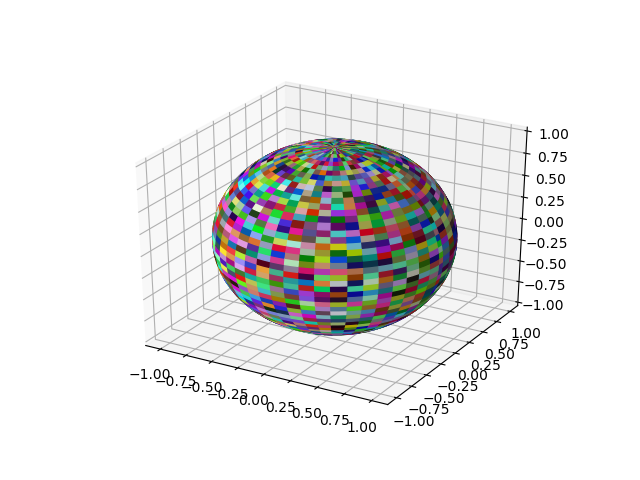Owner Author

gizmaa commented Jun 23, 2017

 @feima0011 The results seem to be inconsistent. Mine looks like this: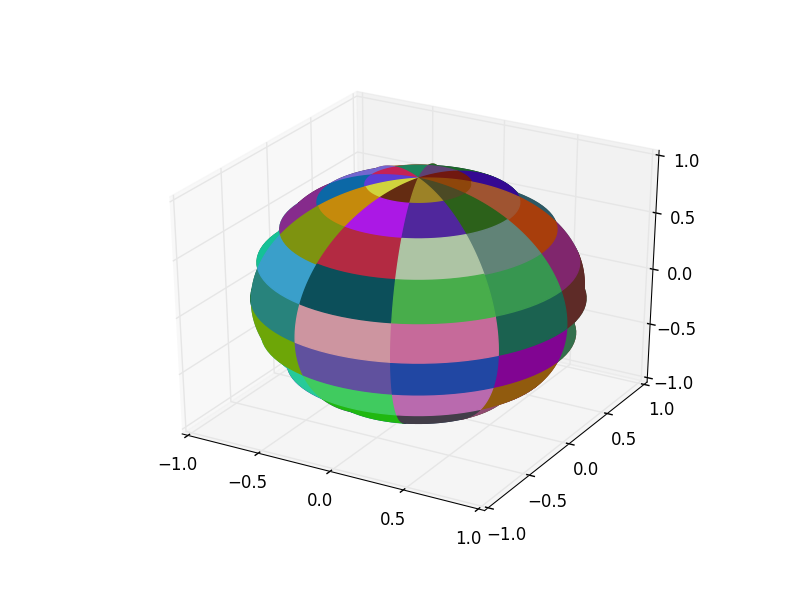Are you using any other special settings? My system: Kubuntu 16.04 (Linux) Julia 0.6.0-rc3.0

feima0011 commented Jun 30, 2017

 @gizmaa NO other special settings , plot more patches, I think you should get the desired results.
Owner Author

mboedigh commented Sep 2, 2017

 You are probably aware. But this doesn't work as written. I personally have no idea how to make it work, because I'm too new to any of this. ax:set_ylim UndefVarError: ax not defined I guess it's far too much trouble to add a line or two to make the examples actually work....
Owner Author

gizmaa commented Sep 29, 2017 • edited

 @mboedigh I'm assuming you're talking about the Python/Julia comparison at the very top. It's not meant to be a full example but a comparison of how to translate Python to Julia. ax = axes() ax[:set_ylim]([-30,10]) An easier way is to just use ylim([-30,10]). Click either link in (IJulia,Code) for fully functional code. This gist is written so that the important bits for each section are quickly and easily visible. All of the rest are in the full example links I've already mentioned. I guess it's far too much trouble to add a line or two to make the examples actually work.... I would also suggest you use a different tone when asking for free help or taking issue with something somebody provides online free of charge. Not everybody will be as kind. Keep in mind Github is collaborative.

davidphysdavalos commented Oct 7, 2017

 Hey, thanks a lot for the useful examples!, by the way, do you have one for vector fields and streamplot?
Owner Author

gizmaa commented Oct 10, 2017

 It's not often that I add examples quickly so give the examples in the matplotlib gallery a try. Most of the time it's just using the command as it would be in Python. The finer points may require a little bit of tweaking but the translation examples are in the first section of this document. If you've created an example and would like it added here just post it somewhere and tell me. When I find time I will clean it up if it needs to be and add it, citing you in the code and snippet. See the "Line Collection" and "3D Surf Plot" examples for how I cite. @davidphysdavalos the quiver (vector field) and streamplot examples have been added.

CarloLucibello commented Oct 15, 2017 • edited

 some code for plotting MNIST images, if of any interest. Showing subplots, imshow, axes removal and tight_layout with negative paddings to remove all interspaces. MLDatasets has not been published to METADATA yet and has to be cloned from here using PyPlot import MLDatasets: MNIST import IterTools: product x, y = MNIST.testdata() nrow, ncol = 4, 4 fig = figure("plot_mnist",figsize=(6,6)) for (i, (c, r)) in enumerate(product(1:ncol, 1:nrow)) subplot(nrow, ncol, i) imshow(x[:,:,i]', cmap="gray") #notice the transpose ax = gca() ax[:xaxis][:set_visible](false) ax[:yaxis][:set_visible](false) end tight_layout(w_pad=-1, h_pad=-1, pad=-0.5)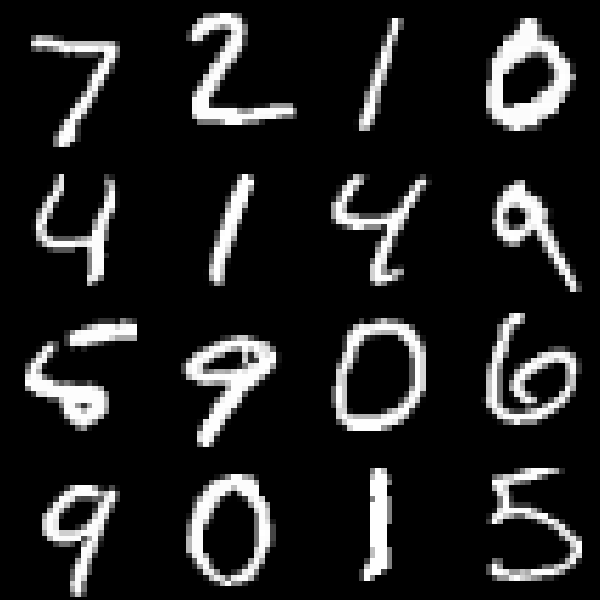mafla commented Feb 23, 2018

 This is an extremely helpful collection of examples! Thank you all! I am plying with this for the first time and have a question, as I don’t fully understand the logic of this yet. How can I make sure that the labels of the colorbar don’t overlap with the ticks? How can I use the set_label_postion to "left"? How can I rotate the labels of my labels (Jan, Feb, …) e.g. with set_rotation? Greetings! using PyPlot fig = figure("polar_lineplot", figsize = (10, 10)) # create a polar axis ax = axes(polar = "true") # define time (all could be done more elegantly in julia I guess... ) time_ang = 1:0.25:13 N_time = length(time_ang) time_ang = time_ang .* (360/12) time_rad = time_ang .* (pi / 180) # create a continous var for the background N_div = N_time*10 N_max = 6 y = linspace(0, N_max, N_div) # grid to fill the polar plot xgrid = repmat(time_rad', N_div, 1) ygrid = repmat(y, 1, N_time) # background to the range of values axsurf = ax[:contourf](xgrid, ygrid, ygrid, N_max, cmap=ColorMap("gray_r"), extend="max") # add colorbar cb = colorbar(axsurf, fraction = 0.05, shrink = 0.5, pad = 0.1) cb[:set_label](label = "Variable [units]", rotation=270) # add your data p = plot(time_rad, rand(length(time_rad)).*3) # set polar ticks and labels month_ang = 0:11 month_ang = month_ang .* (360/12) month_lab = ["Jan"; "Feb"; "Mar"; "Apr"; "May"; "Jun"; "Jul"; "Aug"; "Sep"; "Oct"; "Nov"; "Dez"] ax[:set_thetagrids]([angles = month_ang;], [labels = month_lab;], [frac=1.2;], [rotation = month_ang;],) # set Jan to the top of the plot ax[:set_theta_zero_location]("N") # switch to clockwise ax[:set_theta_direction](-1)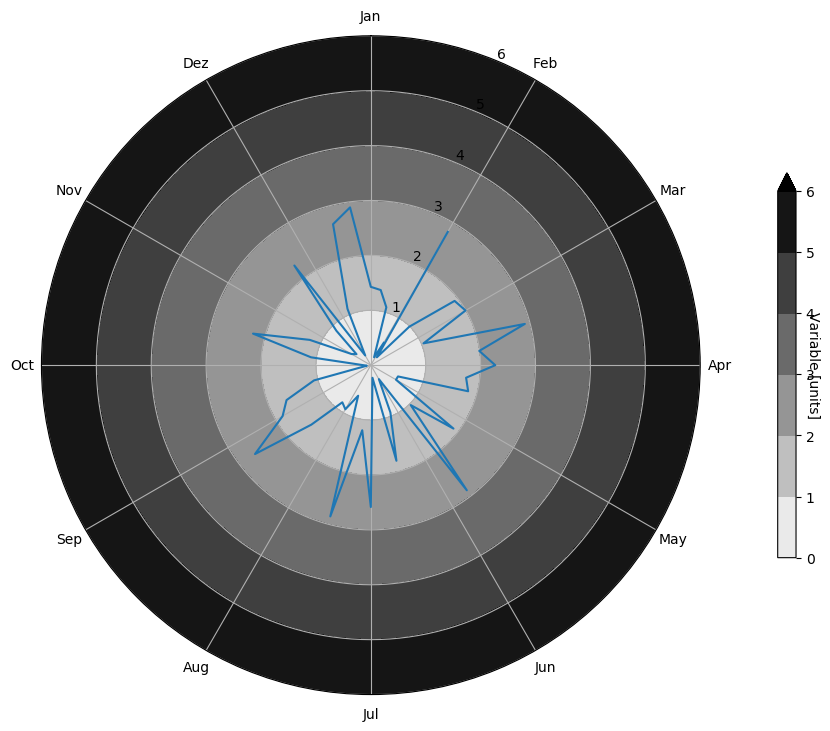Owner Author

gizmaa commented Mar 15, 2018 • edited

 @mafla cb[:set_label]("Variable [units]",labelpad=15) I adjusted this Stack Overflow answer. Hopefully my answer isn't too late for your plot. After more complex plots I would recommend using fig[:canvas][:draw]. Commands may work but not update the plot itself. Edit: forgot the link

samjurl commented May 4, 2018

 Hi, maybe it is a stupid question but how does one manipulate the figure object? For example, if I create a figure f in one cell then plot it, and then wish to zoom (using xlim, ylim) in on regions in different cells, without having to completely re-plot every time?
Owner Author

gizmaa commented May 24, 2018

 @samjurl There are buttons on the figure itself along with keyboard shortcuts to help you navigate. If you aren't seeing them then the first thing to try are the shortcuts. 'o' will let you interactively zoom in and out. 'r' will restore the zoom state to the initial settings. I'm not sure what could cause the controls be nonexistent.

ivemv commented Jul 15, 2018

 Hi Thanks for the examples, very useful! i have a question.., i'm trying to plot three graphics but i want it all for different size and i can't do it yet, some idea to?
Owner Author

gizmaa commented Jul 19, 2018

 @ivemv What exactly do you mean? Do you want three independent plots each with a different size? Do you want one window with three plots each with a different size? Figures are easy. fig1 = figure("First Figure",figsize(4,4)) # Plot something fig2 = figure("Second Figure",figsize=(5,3)) # Plot something fig3 = figure("Third Figure",figsize=(5,10)) # Plot something The second scenario is a little more annoying. fig = figure(figsize=(8,6)) ax1 = axes(position=[.2,.2,.5,.5]) # Plot something ax2 = axes(position=[.8,.1,.1,.8]) # Plot something The position in the axes case is a percentage where the first two numbers are the location of the lower left corner of the axes and the second two are the size. Both sets of numbers are based on the lower left corner of the figure, [0,0] is the lower left corner. ax2 would then start at 80% right along the x-axis, 10% up on the y-axis and be 10% wide and 80% high.

Moelf commented Sep 1, 2018 • edited

 Hist plotting does not work in 1.0; I guess you will have to do something like PyPlot.grid
Owner Author

gizmaa commented Sep 3, 2018

 At the moment I'm really behind in updating to Julia 0.7/1.0. Once work settles down I should have more time to make the updates.

ianshmean commented Feb 7, 2019

 I converted the broken axis in a subplot example from here and thought it would be a helpful example using PyPlot, PyCall @pyimport mpl_toolkits.axes_grid1 as axes_grid1 x = rand(100) y = rand(100) y2 = rand(100).+10 fig, axes = subplots(nrows=2, sharex=true) ax = axes divider = axes_grid1.make_axes_locatable(ax) ax2 = divider[:new_vertical](size="100%", pad=0.1) fig[:add_axes](ax2) ax[:scatter](x, y) ax[:set_ylim](0, 1) ax[:spines]["top"][:set_visible](false) ax2[:scatter](x, y2) ax2[:set_ylim](10, 11) ax2[:tick_params](bottom="off", labelbottom="off") ax2[:spines]["bottom"][:set_visible](false) # From https://matplotlib.org/examples/pylab_examples/broken_axis.html d = 0.015 # how big to make the diagonal lines in axes coordinates ax2[:plot]((-d, +d), (-d, +d), transform=ax2[:transAxes], color="k", clip_on=false,linewidth=0.8) # top-left diagonal ax2[:plot]((1 - d, 1 + d), (-d, +d), transform=ax2[:transAxes], color="k", clip_on=false,linewidth=0.8) # top-right diagonal ax[:plot]((-d, +d), (1 - d, 1 + d), transform=ax[:transAxes], color="k", clip_on=false,linewidth=0.8) # bottom-left diagonal ax[:plot]((1 - d, 1 + d), (1 - d, 1 + d), transform=ax[:transAxes], color="k", clip_on=false,linewidth=0.8) # bottom-right diagonal #create bottom subplot as usual axes[:scatter](x, y)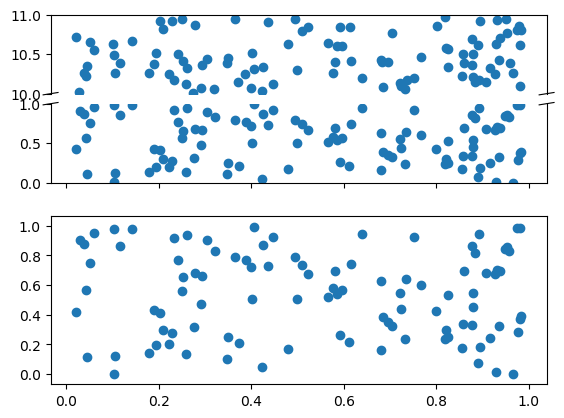gerritgr commented Apr 25, 2019

 Hey, has anyone figured out how to use the surface plot (https://gist.github.com/gizmaa/7214002#surface-and-contour-plots) in Julia 1.x? Thanks
Owner Author

gizmaa commented May 10, 2019

 @gerritgr Everything has finally been updated. Next week I will try and double check everything and add an example or two.